# How to Add Dashes into Numeric String/Phone Number/SSN in Excel

Suppose we have a list of numbers and we want to separate them into different parts by dash, how can we quickly add dashes into the numeric string? This article will provide you two ways to add dashes, one is to add dash by formula, the other one is set number format by custom in format cells. We also provide the method to add dashes for Phone Number and SSN.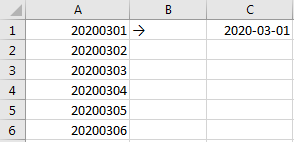In above table, change 20200301 in A1 to 2020-03-01 in C1, we need to add two dashes to separate the string, though we can add dash manually one by one, it is still a little complex for users. So, we need a simple way to add dashes now.

## Method 1: Add Dashes by Formula

Step 1: In C1, enter the formula =TEXT(A1,”????-??-??”). In this formula, ????-??-?? is the final format of string.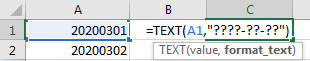Step 2: Click Enter to get result. Verify that numeric string is displayed into three parts and connected by dash properly.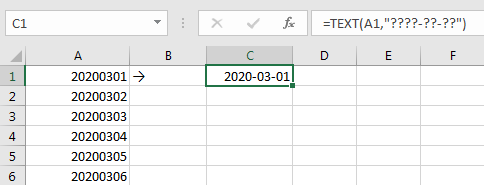Step 3: Drag the fill handle to fill C2 to C6. Verify that all numeric strings are add separated by dashes properly.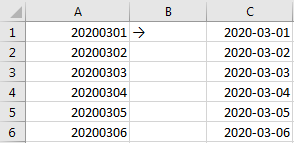Note:

1.You can set format_text parameter depends on your demand. For example, in this case if you enter =TEXT(A1,”??-??-??-??”), then numeric string 20200301 will be displayed as 20-20-03-01 finally.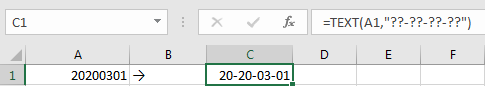2.You can use other formula to set format for string. For example, enter formula =LEFT(A1,4)&”-“&MID(A1,5,2)&”-“&RIGHT(A1,2). Then you can get the same result.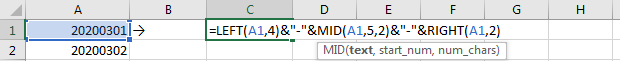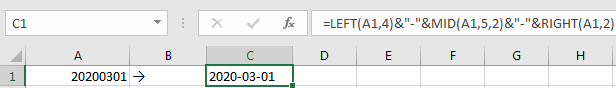## Method 2: Add Dashes by Set Format as Custom in Format Cell

Step 1: Copy A column to C column.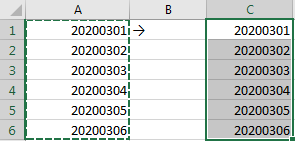Step 2: Select C2:C6, then right click to load menu, select Format Cell.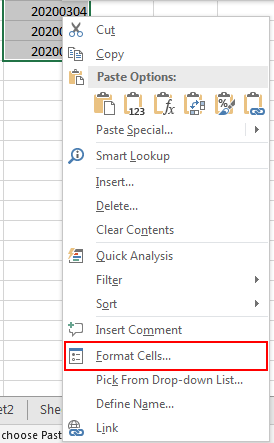Step 3: In Format Cell, under Number tab, select Custom from Category list. Then in Type textbox, enter 0000-00-00. Then after typing the Type, in Sample field, 2020-03-01 is displayed. Then click OK.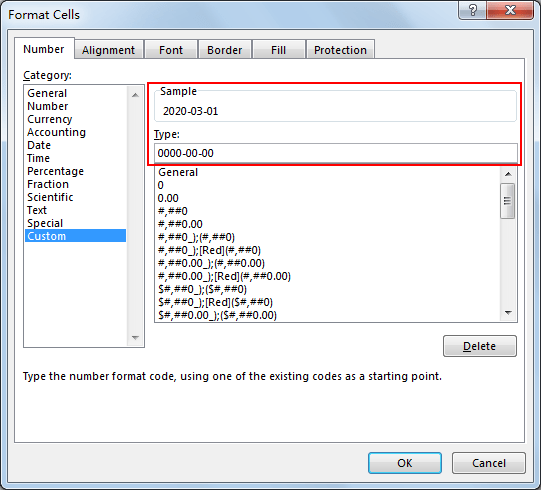Step 4: Verify that dashes are added into string as we expect.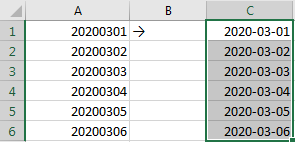## Method 3: Add Dashes by Set Format as Special in Format Cell

If we want to add dashes into number with fixed format like Phone Number or SSN, you can directly select Special->Type->Phone Number (or Social Security Number) in Format Cells, see screenshot below.

Screenshot 1: Set number to Phone Number.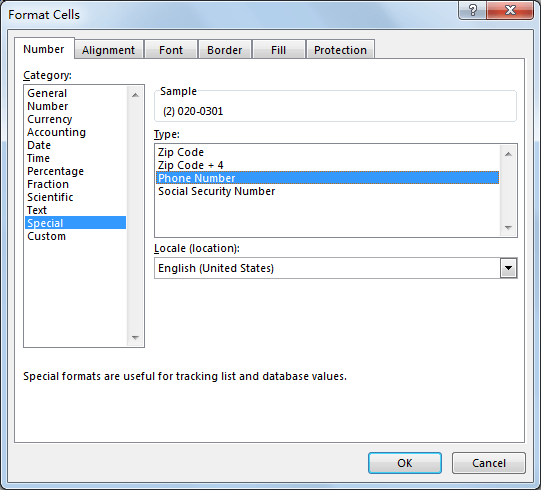Screenshot 2: Set number to Social Security Number.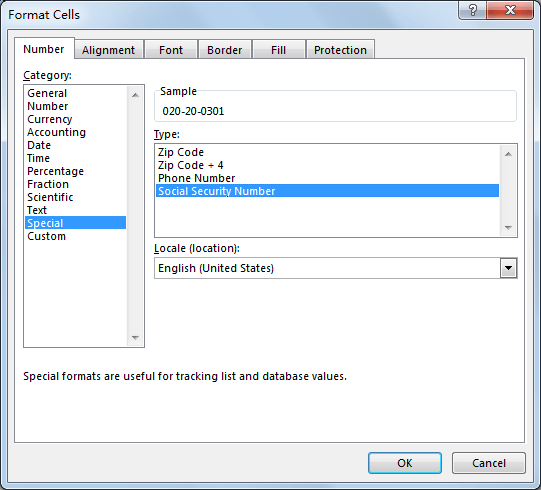Related Posts

Cap percentage values between 0 and 100

This article will talk about how to cap the percentage values between 0% and 100% in Microsoft Excel Spreadsheet or Google Sheets. If you are a newbie on Excel or google Sheets, you may be able to do this by ...

Calculate Cap Percentages to Specific Value

This article will talk about how to limit the cap percentage of a given amount to a given value in Microsoft Excel Spreadsheet or Google Sheets. If you are a newbie on Excel or google Sheets, you may be able ...

Calculate Win Loss Tie

Suppose you got a task to calculate the win, loss, and tie totals; what would you do? If you are new to Ms Excel and don't have enough experience with it, then you might do this task manually but let ...

Calculate Years Between Dates In Ms Excel

If you are an avid Ms Excel user, then you might have come across a task in which you needed to calculate the years between the dates; you might take it easy and do this task manually, which is also ...

Calculate Number of Hours between Two Times

Calculating the difference between two times might be a valuable statistic for subsequent computations or averages, whether you're producing a time sheet for staff or recording personal exercises. While Excel has a plethora of complex functions, including date and time ...

Calculate Loan Interest in Given Year

When you borrow money, you are supposed to repay it gradually. Lenders, on the other hand, want to be compensated for their services and the risk they incur by lending you money. That is, you will not just repay the ...

Calculate Interest Rate for Loan

The interest rate is the fee charged by a lender to a borrower and is expressed as a percentage of the principal—the lent amount. The interest rate on a loan is often expressed as an annual percentage rate, abbreviated as ...

Calculate Interest for Given Period

Using the IPMT function in Excel, we can compute the interest payment on any loan. This step-by-step tutorial will guide Excel users of all skill levels through the process to calculate interest for given period. Finally, the formula: =IPMT(B3/12,1,B5,-B2) The ...

How To Use Excel GCD Function

This post will guide you how to use Excel GCD function with syntax and examples in Microsoft excel. Description The Excel GCD function Returns the greatest common divisor of two or more integers. So you can use the GCD function ...

Calculate A Ratio From Two Numbers In Excel

In elementary mathematics, a ratio is a connection or comparison between two or more integers. For example, ratios are often expressed as ":" to demonstrate the relationship between two numbers. You would think that manually calculating a ratio from two ...

Sidebar